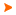Podaci o VU Početna stranicaArhiva nastavnih programaArhiva ak. kalendaraAbbreviation: B11A03 Load: 30(L) + 0(P) + 0(FE) + 0(LE) + 0(S) + 0(PEE) + 30(E) + 0(DE)
 Lecturers in charge: prof. dr. sc. Mario Brkić Lecturers: Course description: Understand and apply the basic laws of geometrical optics, mechanics, oscillatory motion and waves, as well as electromagnetism.Learning outcomes at the level of the programme to which the course contributesUnderstand mathematical methods and physical laws applied in geodesy and geoinformatics.Apply knowledge of mathematics and physics for the purpose of recognizing, formulating and solving of problems in the field of geodesy and geoinformatics.Exercise appropriate judgments on the basis of performed calculation processing and interpretation of data obtained by means of surveying and its results.Take responsibility for continuing academic development in the field of geodesy and geoinformatics, or related disciplines, and for the development of interest in lifelong learning and further professional education.Learning outcomes expected at the level of the courseDerive and apply the equations of geometrical optics.Describe the motion by vectors of position, velocity and acceleration.Apply Newton's laws of motion.Describe the motion of the gyroscopes.Derive and apply the Kepler's laws.Derive the general expression for the gravitational potential energy and define the potential and equipotential surface.Describe and compare the simple and physical pendulum.Describe the harmonic waves.Describe the electric field, electric potential difference, and electric current; describe the magnetic field of a current loop.Describe the electromagnetic induction.Course content broken down in detail by weekly class schedule (syllabus)Physics and measurement. Standards, dimensional analysis, significant digits, order of magnitude, scalars and vectors, unit vectors and vector components, analytical method of addition of vectors, scalar product of vectors, vector product.Geometrical optics. The absorption, reflection and transmission of light rays, the laws of geometrical optics, Huygens' principle, total reflection, reflection, mirror equation, magnification.Optical instruments. Refraction, lens equation, positive and negative lenses, magnification of the lenses, eye, simple magnifier, microscope, telescope.Kinematics 1D. Position and displacement, velocity in 1D, motion with constant velocity, acceleration in 1D, motion with constant acceleration, the area under the graph of v(t) and a(t), free-fall.Kinematics 2D. Position, velocity and acceleration in 2D, uniform circular motion, tangential and radial acceleration; position, velocity and acceleration in 3D.Newton's laws of motion. Newton's first law, inertial reference systems, Newton's second law, Newton's third law, gravitational force and weight, contact forces. Applications of Newton's Laws. Motion in accelerated frames.Work and energy. Work done by constant and varying force, Hooke's law, general expression for the work, kinetic energy and the work, work of conservative forces, potential energy of a system, conservation of mechanical energy, relationship between conservative forces and potential energy, power. The center of mass, motion of a system of particles.Rotational kinematics. Angular position, angular velocity and angular acceleration, rotational motion with constant angular acceleration, angular and linear quantities, rotational kinetic energy, moment of inertia, parallel axis theorem.Rotational dynamics. Torque and angular acceleration, angular momentum, angular momentum of a rotating rigid object, conservation of angular momentum, a variable moment of inertia of the system, motion of gyroscopes; the conditions of static equilibrium, center of gravity.Newton's law of universal gravitation. Law of gravitation for particles, gravitational force between the bodies, variation of free-fall acceleration at the surface of the Earth, gravitational field, gravitational flux, gravitational potential energy, Kepler's laws.Oscillations and waves. Kinematics of simple harmonic motion, dynamics of simple harmonic motion, simple pendulum, physical pendulum, traveling waves, the wave function, interference of harmonic waves, harmonic wave, constructive and destructive interference, Doppler effect.The electric current. Electric charge, Coulomb's law and electric field, Gauss's law for electric field, electric potential energy, electric potential and potential difference, electric current, Ohm's law, resistance, Joule's law, batteries.The magnetic field. Sources of the magnetic field, magnetic force acting on a current-carrying conductor, torque on a current loop in a uniform magnetic field, magnetic moment, Biot-Savart law, the magnetic field current through a long straight wire, magnetic field on the axis of a circular current loop, Ampere's law, Gauss's law for the magnetic field.Electromagnetic induction. Faraday's law of induction, Lenz's law, generators.Screening student workClass attendance - 1 ECTSTests - 2 ECTSOral exam - 2 ECTSWritten exam - 2 ECTS
 Lecture languages: - - -
 Compulsory literature: 1. Temeljna literatura obuhvaća skripta, vježbe, domaće zadaće i numeričke vježbe (http://e-ucenje.geof.unizg.hr/).
 Recommended literature: 2. Serway, Jewett: Physics for Scientists and Engineers, te pripadni Solutions Manual. 3. Benčić, Solarić: Mjerni instrumenti i sustavi u geodeziji i geoinformatici.Legend L - Lectures P - Practicum FE - Field exercises LE - Laboratory exercises S - Seminar PEE - Physical education excercises. E - Exercises DE - Design exercises * - Not graded| Početna stranica | Visoka učilišta | Korisničke stranice | | Na vrh | Copyright (c) 2006. Ministarstva znanosti, obrazovanja i športa. Sva prava zadržana. Programska podrška (c) 2006. Fakultet elektrotehnike i računarstva. Oblikovanje(c) 2006. Listopad Web Studio. Posljednja izmjena 2018-10-09